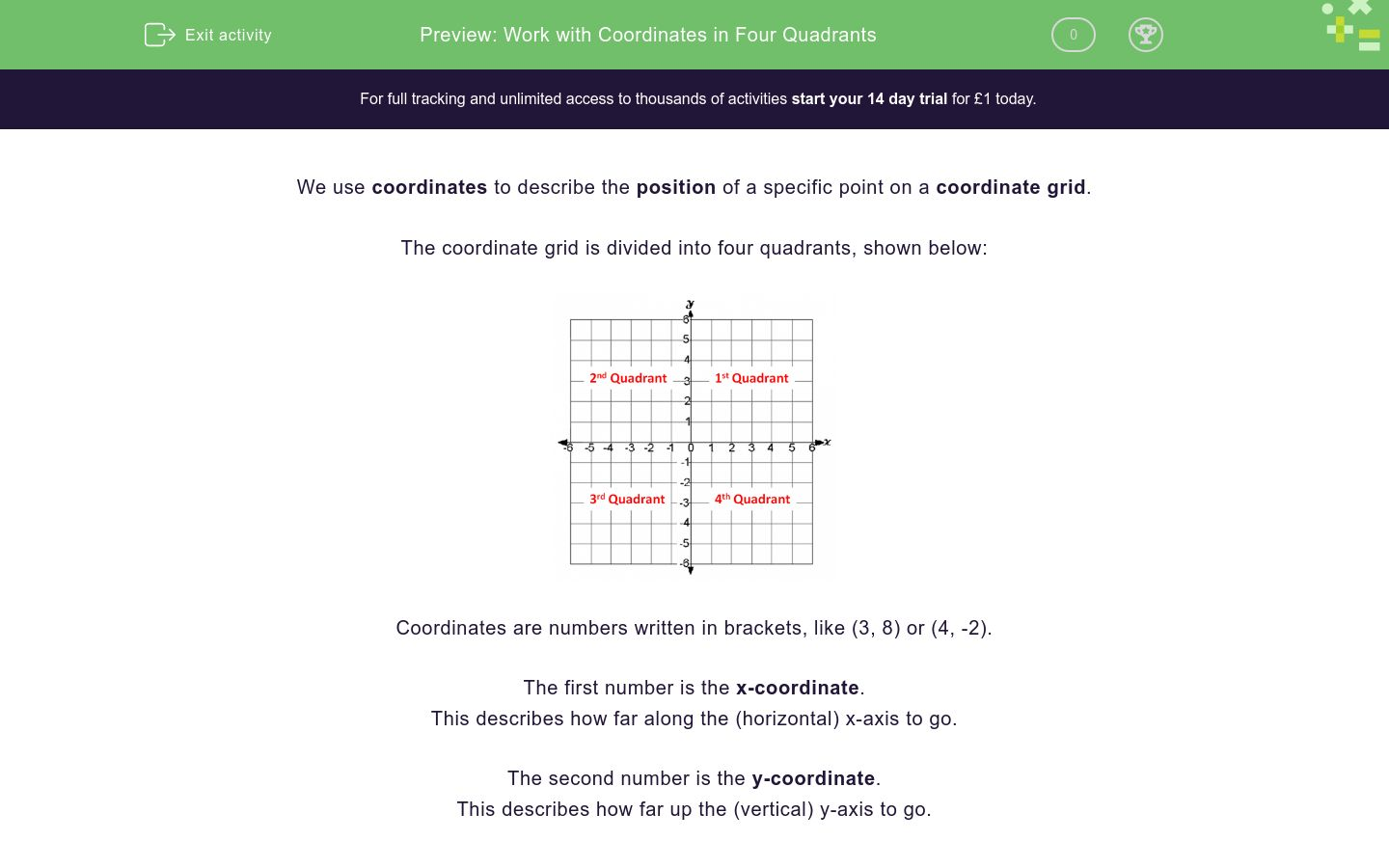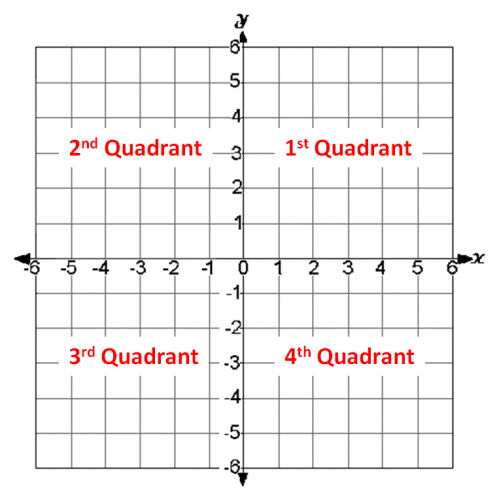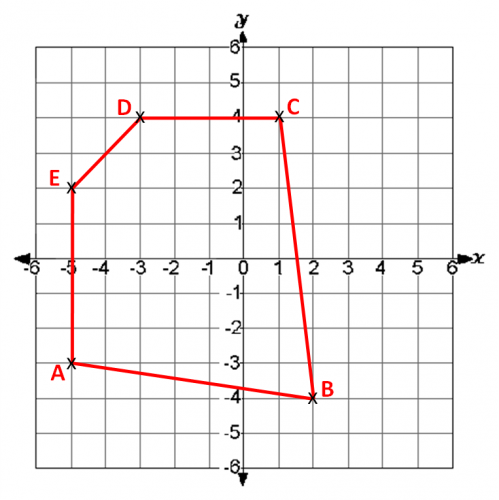# Work with Coordinates in Four Quadrants

In this worksheet, students will identify the quadrant location of specific coordinates on the coordinate grid and solve problems involving coordinates using both negative and positive values for x and y coordinates.Key stage:  KS 4

GCSE Subjects:   Maths

GCSE Boards:   Pearson Edexcel, OCR, Eduqas, AQA

Curriculum topic:   Algebra, Graphs of Equations and Functions

Curriculum subtopic:   Graphs, Graphs of Equations and Functions

Difficulty level:### QUESTION 1 of 10

We use coordinates to describe the position of a specific point on a coordinate grid.

The coordinate grid is divided into four quadrants, shown below:Coordinates are numbers written in brackets, like (3, 8) or (4, -2).

The first number is the x-coordinate.

This describes how far along the (horizontal) x-axis to go.

The second number is the y-coordinate.

This describes how far up the (vertical) y-axis to go.

e.g. Look at the points shown on the coordinate grid below.

K has coordinates (1, 4). N has coordinates (-3, 1). F has coordinates (-3, -3) and P has coordinates (1, -3).Where both the x- and y-coordinates are positive, the coordinate will appear in the 1st Quadrant

Where the x-coordinate is negative but the y-coordinate is positive, the coordinate will appear in the 2nd Quadrant

Where both the x- and y-coordinates are negative, the coordinate will appear in the 3rd Quadrant

Where the x-coordinate is positive but the y-coordinate is negative, the coordinate will appear in the 4th QuadrantIn this activity, we will identify the quadrant location of specific coordinates and solve problems involving coordinates using both negative and positive values for the x- and y-coordinates.

In which quadrant of the coordinate grid is point (5, -2) located?

First

Second

Third

Fourth

In which quadrant of the coordinate grid is point (-2, 3) located?

First

Second

Third

Fourth

Look at the grid below.Which quadrant is point A in?

First

Second

Third

Fourth

Look at the grid below.Match each lettered point to its correct pair of coordinates.

## Column B

A
(-2, 5)
D
(-5, -3)
E
(0, 1)
G
(6, -3)
I
(3, -2)
J
(3, 5)

Consider the grid below.Choose whether the pairs of points below have the same value as their x-coordinates.

Consider the grid below.Choose whether the pairs of points below have the same value as their y-coordinates.

Tick all of the correct statements about coordinate grids from the list below.

Both values of coordinates in the third quadrant are negative

It doesn't matter which axes you use first when plotting coordinates

Points cannot have the same value for the x- and the y-coordinates

Point (0, 5) lies exactly on the y-axis

On the grid below you can see an irregular pentagon with vertices labelled A, B, C, D and E.Choose the combination of coordinates which accurately matches the corners of this shape.

A (-3, -5), B (-4, -2), C(4, 1), D (4, -3), E (2, -5)

A (-5, -3), B (-2, -4), C(1, 4), D (-3, 4), E (-5, 2)

A (-5, -3), B (-2, -4), C (-5, 2), D (-3, 4), E (1, 4)

A (-3, 4), B (-2, -4), C (-5, 2), D (-5, -3), E (1, 4)

Consider the grid below.Then decide whether each statement listed is true or false.

Look at the grid below.Match each pair of coordinates below to the letter that accurately shows their location on the grid.

## Column B

(0, -2)
D
(-2, 0)
P
(-3, 1)
H
(1, -3)
N
• Question 1

In which quadrant of the coordinate grid is point (5, -2) located?

Fourth
EDDIE SAYS
(5, -3) is located in the 4th quadrant (bottom, right) as its x-coordinate is positive and its y-coordinate is negative.• Question 2

In which quadrant of the coordinate grid is point (-2, 3) located?

Second
EDDIE SAYS
(-2, 3) is in the 2nd quadrant (top, left). This is because its x-coordinate value is negative but its y-coordinate value is positive.
• Question 3

Look at the grid below.Which quadrant is point A in?

Third
EDDIE SAYS
Point A is in the 3rd quadrant. The 1st quadrant is in the top right, the 2nd is in the top left, the 3rd in the bottom left, and the 4th quadrant in the bottom right. Remember that we can look for the visual location of a point on the coordinate grid (if shown) or whether the values of the x- and y-coordinate are positive or negative. Can you work out the coordinates of point A independently?
• Question 4

Look at the grid below.Match each lettered point to its correct pair of coordinates.

## Column B

A
(-5, -3)
D
(0, 1)
E
(3, -2)
G
(6, -3)
I
(3, 5)
J
(-2, 5)
EDDIE SAYS
Remember that the first number in the pair of coordinates tells us where to go horizontally (across) and the second number tells us where to go vertically (up/down). Points B, D and I are in the first quadrant, so will contain positive values for both their x- and y-coordinates. Points C and J are in the second quadrant. so will have a positive y-coordinate and a negative x-coordinate. Points A and F will have both negative x- and y-coordinates, as they are in the third quadrant of the coordinate grid. Finally, points E, G and H are in the fourth quadrant. so will have a positive x-coordinate and a negative y-coordinate. Can you find the matches now?
• Question 5

Consider the grid below.Choose whether the pairs of points below have the same value as their x-coordinates.

EDDIE SAYS
This question is asking us to consider if the pairs listed have the same value for their x-coordinate, i.e. the same first number in their coordinates. The only pair of points with the same value as their x-coordinate is F (-3, 3) and H (-3, 5). All the other pairs are different e.g. A (-5, -3) and B (-1, -3). Be careful not to confuse the x- and y-coordinates here.
• Question 6

Consider the grid below.Choose whether the pairs of points below have the same value as their y-coordinates.

EDDIE SAYS
The pairs of points with the same value as their y-coordinate are: A (-5, -3) and B (-1, -3); B (-1, -3) and C (3, -3); G (2, 3) and F (-3, 3)
• Question 7

Tick all of the correct statements about coordinate grids from the list below.

Both values of coordinates in the third quadrant are negative
Point (0, 5) lies exactly on the y-axis
EDDIE SAYS
Two of these statements are true and two are false. In the 3rd quadrant, both the x- and y-coordinates must be negative e.g. (-2, -5). If they are not both negative, then a point will not appear in the 3rd quadrant. When plotting coordinates, we must always go along and then up, so this statement is false and incorrect. It is definitely possible for points to have the same value for their x- and y-coordinates so this statement is also untrue e.g. (2, 2). Finally, point (0, 5) does lie on the y-axis. We go along 0 units across and up 5, so the point will be positioned exactly on the vertical axis.
• Question 8

On the grid below you can see an irregular pentagon with vertices labelled A, B, C, D and E.Choose the combination of coordinates which accurately matches the corners of this shape.

A (-5, -3), B (-2, -4), C(1, 4), D (-3, 4), E (-5, 2)
EDDIE SAYS
Work through each point one at a time. How many squares along the x axis do you need to travel? How many squares up or down the y axis do you need to travel? The correct answer is: A (-5, -3) B (-2, -4) C(1, 4) D (-3, 4) E (-5, 2) The first option looks very similar, but the x- and y- coordinates are the wrong way round! This is a very common mistake to make, particularly if you are working quickly.
• Question 9

Consider the grid below.Then decide whether each statement listed is true or false.

EDDIE SAYS
Work through each statement one at a time, referring to the grid above to verify if it is accurate or not. The first quadrant contains 2 points only, not 3. There are 2 points in the third quadrant, so this is the only true statement. Point G is located in the fourth quadrant, not the second. Point D lies on the y-axis, not the x-axis. Hopefully none of these sneaky statements tripped you up!
• Question 10

Look at the grid below.Match each pair of coordinates below to the letter that accurately shows their location on the grid.

## Column B

(0, -2)
H
(-2, 0)
D
(-3, 1)
N
(1, -3)
P
EDDIE SAYS
Some of these look very similar but remember that the first number is the horizontal distance from the origin (x) and the second number is the vertical distance (y). Take your time to locate how many squares to go along and then up or down for each pair. Congratulations on completing this activity! Why not move on to another activity in our algebraic graphs section now that you are confident with coordinates?
---- OR ----

Sign up for a £1 trial so you can track and measure your child's progress on this activity.

### What is EdPlace?

We're your National Curriculum aligned online education content provider helping each child succeed in English, maths and science from year 1 to GCSE. With an EdPlace account you’ll be able to track and measure progress, helping each child achieve their best. We build confidence and attainment by personalising each child’s learning at a level that suits them.

Get started